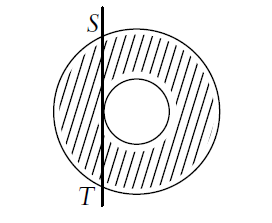# Is this enough?

Geometry Level 2In the above diagram, the line ST is tangent to the smaller of two concentric circles, and is 36 cm long. The area of the annulus (shaded region) can be written as $a\pi$.

What is the value of $a$?

×